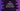# Find if a number is prime or not in C++# Introduction :

This tutorial will show you how to verify if a number is prime or not in C++ programmatically. The program will ask the user to enter a number, it will check if it is prime or not and print out the result.

This is a beginner level tutorial and it will give you the basic overview of how to read user inputs, how to print data to the user, how to use a function and how to use a loop in C++.

## Prime numbers :

Prime numbers are natural numbers greater than 1 with factors only 1 and the number itself. For example, 2, 3, 5, 7, 11, 13 etc. are prime numbers. If a number is not prime, it is called a composite number.

To find out if a number is prime or not, we can divide it by all numbers starting from 2. Actually, we need to divide it from 2 to number/2. Because any number greater than number/2 can’t be a factor of that number. We are going to implement this same logic in our program below:

## C++ program :

``````#include <iostream>
using namespace std;

//1

//2
int main()
{
//3
int n;

//4
cout << "Enter a number to check for prime : "; cin >> n;

//5
cout << "=> " << n << " is a prime number" << endl;
else
cout << "=> " << n << " is not a prime number" << endl;

return 0;
}

//6
{
//7
for (int i = 2; i <= number / 2; i++)
{
//8
if (number % i == 0)
{
return false;
}
}
//9
return true;
}``````

### Explanation :

The commented numbers in the above program denote the step numbers below :

1. isPrimeNumber is a method that takes one integer number as the argument and returns one boolean value. We have the implementation of this method at the end of the program but we need to declare it as we are using it in the main method. The main method runs at the start of the program and we are using isPrimeNumber in it. The initial declaration will tell the compiler that we have one method with name isPrimeNumber available in this program.
2. main() runs at the start of the program.
3. Create one integer variable n to hold the user input number.
4. Ask the user to enter a number. Read it and store it in variable n. cout is used to print a message and cin is used to read input in C++.
5. Check if the number is prime or not using an if-else block. This block will call the isPrimeNumber method with n as the argument first. If the return value of this method is true, it will execute the code block in the if statement. Else, it will execute the else statement block. If it is a prime number, if block will run and if it is not, else block will run.
6. This is the implementation of the isPrimeNumber method. As you can see here, we have the implementation at the end of the program.
7. Using a for loop, check for all numbers starting 2 to number/2 if it can divide the given number or not.
8. Using % symbol, we are checking the remainder of the division i.e. if the remainder is 0, the current value of i is a factor of number. If it is a factor, return false. It will stop the execution of this program at this point.
9. If the for loop doesn’t find any factors, it will move to step 9 and return true, i.e. it is a prime number.

### Sample Output :

``````Enter a number to check for prime : 13
=> 13 is a prime number

Enter a number to check for prime : 12
=> 12 is not a prime number

Enter a number to check for prime : 5
=> 5 is a prime number``````

### Conclusion :

In this C++ tutorial, we learned how to find if a number is prime or not. Try to run the program and drop one comment below if you have any queries.